Chess

How many different ways can initiate a game of chess (first pass)?

Result

n =  400

Solution:Leave us a comment of this math problem and its solution (i.e. if it is still somewhat unclear...):Be the first to comment!To solve this verbal math problem are needed these knowledge from mathematics:

See also our variations calculator. Would you like to compute count of combinations?

Next similar math problems:

1. Chess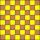How many ways can select 4 fields on classic chess board with 64 fields, so that fields don't has the same color?
2. Cars plates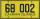How many different licence plates can country have, given that they use 3 letters followed by 3 digits?
3. Trainings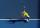The table contains tennis training schedule for Saturday's younger students during the winter indoor season. Before the start of the summer season is preparing a new training schedule. Tomas Kucera will be able to practice only in the morning, sisters Kova
4. MetalsIn the Hockey World Cup play eight teams, determine how many ways can they win gold, silver and bronze medals.
5. MedalsIn how many ways can be divided gold, silver and bronze medal among 21 contestant?
6. Olympics metalsIn how many ways can be win six athletes medal positions in the Olympics? Metal color matters.
7. Sweater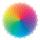Dana confuses sweater and wool has a choice of seven colors. In how many ways she can choose from three colors to the sleeves?
8. Logik game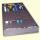Letter game Logik is a two player game, which has the following rules: 1. The first player thinks five-letter word in which no letter is not repeated. 2. The second player writes a five-letter word. 3. The first player answers two numbers - the first numbe
9. Seven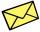Seven friends agree to send everyone a holiday card. How many postcards were sent?
10. Coin and die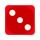Flip a coin and then roll a six-sided die. How many possible combinations are there?
11. PIN - codesHow many five-digit PIN - code can we create using the even numbers?
12. Glass with icecream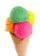We have 6 kinds of ice cream and 5 kinds of fruit. We put 3 cups of ice cream and 2 fruits into each glass. How many can unique decorated glasses be?
13. Tricolors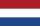From the colors - red, blue, green, black and white, create all possible tricolors.
14. Task of the year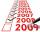Determine the number of integers from 1 to 106 with ending four digits 2006.
15. VariationsDetermine the number of items when the count of variations of fourth class without repeating is 42 times larger than the count of variations of third class without repetition.
16. One threeWe throw two dice. What is the probability that max one three falls?
17. Theorem proveWe want to prove the sentence: If the natural number n is divisible by six, then n is divisible by three. From what assumption we started?